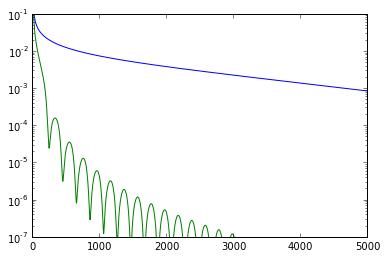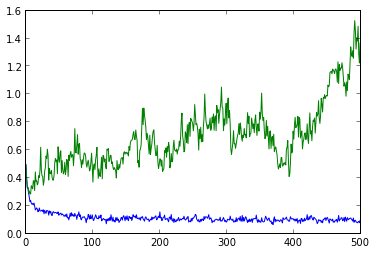# Noise tolerance of accelerated gradient descent¶

Here we compare the noise tolerance of basic gradient descent with that of accelerated gradient descent for smooth convex optimization. We will see that errors in the gradient accumulate for accelerated gradient descent, while they do not accumulate for basic gradient descent. This illustrates that for noisy convex optimization basic gradient descent is preferable to accelerated gradient descent despite the slower convergence rate in the noise free setting.

In :
import matplotlib.pyplot as plt
import numpy as np

""" Gradient descent for smooth convex function """
x = x0
xs = [x0]
for t in range(0,n_iterations):
xs.append(x)
return xs

""" Accelerated gradient descent for smooth convex function
"""
x = x0
y = x0
xs = [x0]
a = 0
for t in range(0,n_iterations):
a = 0.5 * (1.0 + np.sqrt(1+4.0*a**2))
a2 = 0.5 * (1.0 + np.sqrt(1+4.0*a**2))
gamma = (1.0-a)/a2
y2 = x - (1.0/smoothness) * gradient(x)
x = (1.0-gamma)*y2 + gamma*y
y = y2
xs.append(x)
return xs


## Laplacian of a cycle¶

Below the matrix P defines the Laplacian of a cycle graph and we solve a linear system defined by the Laplacian. This example is typically shown as a tight example for accelerated gradient descent.

In :
n = 100
A = np.zeros((n,n))
for i in range(1,n-1):
A[i,i+1] = 1
A[i,i-1] = 1
A[0,1] = 1
A[n-1,n-2] = 1
P = 2.0*np.eye(n) - A
b = np.zeros(n)
b = 1
opt = np.dot(np.linalg.pinv(P),b)

def path(x):
return 0.5*np.dot(x,np.dot(P,x)) - np.dot(x,b)

return np.dot(P,x) - b

its = 5000

ys3 = [ abs(path(xs3[i])-path(opt)) for i in range(0,its) ]
ys3acc = [ abs(path(xs3acc[i])-path(opt)) for i in range(0,its) ]

plt.yscale('log')
plt.ylim(0,0.1)
plt.plot(range(0,its),ys3,range(0,its),ys3acc)

Out:
[<matplotlib.lines.Line2D at 0x1ba16950>,
<matplotlib.lines.Line2D at 0x1ad2f450>]## Noisy cycle¶

We repeat the same example, but now we had gaussian noise to the gradient. The plot illustrates that accelerated gradient descent diverges, while basic gradient descent converges essentially to the best possible answer given the noise in the gradient.

In :
def noisygrad(x):
return np.dot(P,x) - b + np.random.normal(0,0.1,(n))

its = 500


[<matplotlib.lines.Line2D at 0x1accd550>,
<matplotlib.lines.Line2D at 0x1a38cb50>]